2008-11-14:

## .S.k.y.

c++:gfx:code:easy
About a half year ago I decided that I need an animated (as in "generated realtime") desktop wallpaper.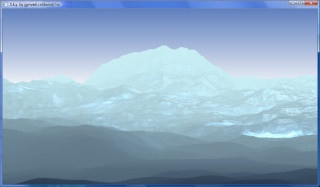I thought it should not use 3D acceleration (no OpenGL/D3D), the FPS should not be to high (2-3 frames per seconds were totally fine with me), and if possible, it should use more then one core (up to 4). I've started to write code, and, as always, didn't finish it. However, something does show on the screen, and imho it ain't all bad, so I decided to write a little on what is it, and how does it work - maybe someone will find it interesting ;> (the images are clickable, except the heightmap; the code for Windows/Mac/Linux and a short video is available at the bottom of the post).

At the left side of the screen there is (should be) a screenshot of an image generated by the app (the terrain does not move, only the lights do). Just in case someone does not recognize what it is (or, what is should be), it's a piece of ice-like, not-textured, terrain with a piece of sky. I'll just add the in the current version of the app the mouse pointer is the source of the light (well, more accurate is to say that the light source is bound to the mouse pointer, because it's a little above the mouse pointer in reality). And now, an illustrated guide to making such an app.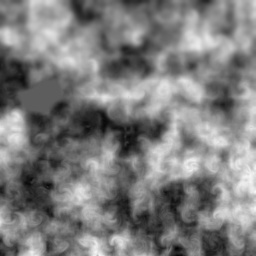The base of everything is a 4097x4097 pixel heightmap (see the thumbnail on the right, the full-size version is in the source pack as a 4097x4097x8 RAW file; the size of the heightmap is related to my desktop size - 3200x1200 (dual head 2x1600x1200)). The heightmap is rendered into 3D using a funny method based on drawing vertical 1-pixel-wide vertical bars, whose size is determined by the value at the specific point of the heightmap. This process is started at the upper left corner of the screen/heightmap, and goes to the end of the row, and then to the next one, and so on. The bare bottom (base) is at the same point as is the point in the heightmap - so the bars basically overlap each other. The following image might clear things out: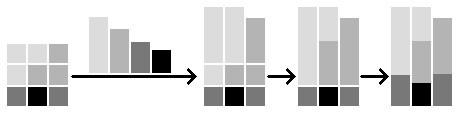As one may see, the heightmap is a bit blurred at the top, and more sharp at the bottom. The objective of this blur is to simulate the details-vanishing-with-distance-increase effect. It could be of course done by code (it's just a Gauss blur with increasing radius), but I guessed it's OK to do it manually.

The heightmap is transformed one more time before it's finally rendered. The objective of the final transformation is to add perspective, and it's again, quite simple. It works this way - the bars that are deepest (the once drawn first) have the lowest height ratio factor, and the closest bars have the heights ratio factor. This is calculated in the MakePicture() function by the following code:
`  for(z = 0; z < HMAP_DEPTH; z++)  {    float mody = powf((float)(1 + HMAP_DEPTH - z), 0.1);    for(x = 0; x < HMAP_WIDTH; x++)      hmap[x + z * HMAP_WIDTH] /= mody;  }`
The hmap array contains of course the heightmap. To mark the depth I've used 'z' instead of 'y'. HMAP_DEPTH and HMAP_WIDTH is the size of the heightmap (4097).
If this transformation would not be used, the terrain would look like the one on the left.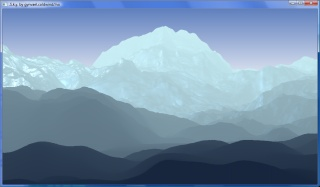The perspective factor is a matter of taste actually, and imho both images (with and without the fix) look OK ("in certain light that is").

The next step is to calculate the normals (vectors showing which way is the "side of a rock" pointed). I've cheated a little here, and used a formula to calculate normal vector for a triangle, loosing some precision in the process (since one "side of a rock" is made of 4 points, and I use just 3). The normals are calculated using the data from the heightmap (X, the point height, and Z), after applying the perspective fix, for every 2x2 quad on the heightmap (which is about the same as 'for every pixel on the heightmap'). The following code is responsible for it:
`  // Make a triangle  float max_y_prev = {    hmap[(x - 0) + (z - 0) * HMAP_WIDTH],    hmap[(x - 1) + (z - 0) * HMAP_WIDTH],    hmap[(x - 0) + (z - 1) * HMAP_WIDTH]  };          Vector3D vp_all = {    Vector3D( (float)x, (float)max_y_prev, (float)z ),    Vector3D( (float)(x-1), (float)max_y_prev, (float)z ),    Vector3D( (float)x, (float)max_y_prev, (float)(z-1) )  };    // Calc normal for that triangle  // XXX the correct way should have two triangles with some avg. normal, but who cares ;p  PreNormalMap[x + z * HMAP_WIDTH] = calc_normal(vp_all);  [...]  Vector3D calc_normal(Vector3D v){  Vector3D out = Vector3D(0,0,0);  Vector3D v1, v2;  // Do some math  v1 = v - v;  v2 = v - v;  out.x = v1.y*v2.z - v1.z*v2.y;  out.y = v1.z*v2.x - v1.x*v2.z;  out.z = v1.x*v2.y - v1.y*v2.x;  // Normalize  out.Norm();  // Done  return out;}`
The outcome can bee seen on the right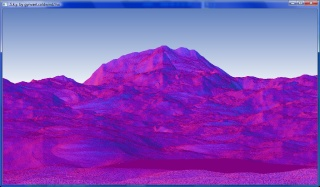(the normal vector is as usual shown as XYZ->RGB). After zooming the image, one will notice the overall noisiness of the picture - this is the results of using a method that takes into consideration just 3 points, where is should use more points (16?). To remove the noise I use a standard blur 3x3->1x1. The blur is coded in the same function (MakePicture()). The code for every pixel looks like this:
`  // This is a simple blur and nothing more  NormalMap[x + z * HMAP_WIDTH] = (    PreNormalMap[x - 1 + z * HMAP_WIDTH] +    PreNormalMap[x     + z * HMAP_WIDTH] +    PreNormalMap[x + 1 + z * HMAP_WIDTH] +    PreNormalMap[x - 1 + (z + 1) * HMAP_WIDTH] +    PreNormalMap[x     + (z + 1) * HMAP_WIDTH] +    PreNormalMap[x + 1 + (z + 1) * HMAP_WIDTH] +    PreNormalMap[x - 1 + (z - 1) * HMAP_WIDTH] +    PreNormalMap[x     + (z - 1) * HMAP_WIDTH] +    PreNormalMap[x + 1 + (z - 1) * HMAP_WIDTH]) * (1.0f / 9.0f);`
The normal map after the blur looks better (see the image on the left).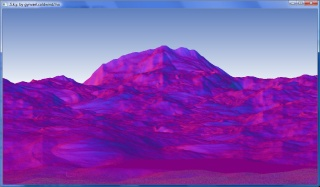Hence the normal calculation take a while, they are cached to a file normal.cache at first run (it weights about 200mb).

Now back to the bars. Since drawing a bar takes longer then drawing a pixel (no surprise here), I could not draw bars in real-time. However, some precalculating here can be also made. Drawing bars of position indexes instead of colors will result in creating a translation array screen-%gt;heightmap. This is the last thing done in the MakePicture function, and the implementation looks like this (this is done for each point of the height map):
`  // Get the heightmap data  float max_y = hmap[x + z * HMAP_WIDTH];    // Bar top and bottom  int y_from = (HEIGHT - 1) - (int)base_y + 100;  int y_to   = (HEIGHT - 1) - (int)base_y - (int)max_y + 100;  // Does reach out of the screen ? Fir it then  if(y_from < 0)          y_from = 0;  if(y_to   < 0)          y_to   = 0;  if(y_from > HEIGHT - 1) y_from = HEIGHT - 1;  if(y_to   > HEIGHT - 1) y_to   = HEIGHT - 1;  // Lift the minimal y ?  if(y_to < total_min_y)    total_min_y = y_to; // Yep  // "Draw" the bar  for(y = y_from; y > y_to; y--)  {    RenderCache[x + y * WIDTH] = x + z * HMAP_WIDTH;  }`
The calculation of minimal value of y is another speed up, that says "don't waste time on rows that don't exists" - it's used to change the limit of final drawing rectangle's top side from 0 to minimal y.

And now a short break for a brief note on the rendering architecture of the application. The described above MakePicture() function is run just once at the beginning of the program, even before the window is created. The rest of the program is kinda interactive, and it's run in a loop. Since the pixel colors do not depend on each other (each pixel color is calculated separately in a pixel-shader-like function), the whole operation can be easily divided into parts that are executed in different threads. In my case I use 4 threads (since I've got a quad CPU in my desktop), where each thread renders a quarter of the screen (the screen is divided horizontally of course). Apart from the renderer threads, there are also two other low-duty threads. The first one coordinates the renderer threads (it mainly sleeps, and when it wakes up, it flips the screen using SDL_Flip()) and the second one handles the mouse/keyboard/window/etc events.
As far as "what is what" goes... the Render() function is the final "pixel shader" (software pixel shader of course), RenderWorker() basically just calls Render() and handles thread communication/synchronizing ('comm/sync' are overstatements). The RenderProc() draws the sky (just once, not in every frame), and synchronizes the renderer threads, sleeps, and calls SDL_Flip(). The last function is main() which at the end has an event handling function.

A half of a sentence on the sky - it's just an old-boring gradient, no dynamic calculation of sky color are present. The code the ddraws the gradient looks like this:
`  // Generate background  //  float bg_start = { 32.0f, 69.0f, 113.0f };  float bg_start = { 96.0f, 128.0f, 190.0f };  //float bg_end   = { 168.0f, 228.0f, 236.0f };  float bg_end   = { 255.0f, 255.0f, 255.0f };  float bg_diff  = { (bg_end - bg_start) / (float)(HEIGHT*18/40),                        (bg_end - bg_start) / (float)(HEIGHT*18/40),                        (bg_end - bg_start) / (float)(HEIGHT*18/40) };  // Render the background (it has to be done only once)  for(y = 0; y < HEIGHT; y++, bg_start += bg_diff, bg_start += bg_diff, bg_start += bg_diff )  {    for(x = 0; x < WIDTH; x++)    {      img[x + y * WIDTH].b = (unsigned char)bg_start;      img[x + y * WIDTH].g = (unsigned char)bg_start;      img[x + y * WIDTH].r = (unsigned char)bg_start;    }  }`
It's pretty simple, so I'll skip the description.

Time to describe the "pixel shader". The final effect consists of 3 things:
1) a "fog" that is a function of distance between the observer and a point on the heightmap, and something that could be called an 'ambient light'
2) diffusive light
3) specular light
Both lights, when in comes to the formula, are a little cheated - I've changed the formula a few times during testing, because the effect wasn't as good as I've expected with the standard formulas.
First, the background color (a simple interpolation of two colors at fog start and end) and the vector from the light to the pixel is calculated. Then a dot product is calculated, and finally, the lights are. The dot product results can be shown using gray scale images. The images below show the dot product when the light source is high left and low right: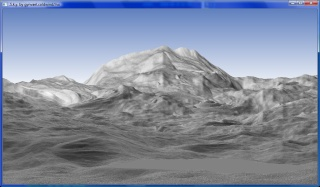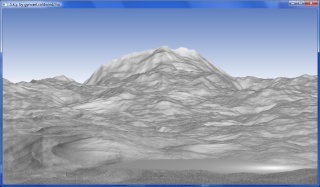The final code responsible for calculating the final color for each pixel looks like this (on the right there are some images: 1st is fog + ambient, 2nd is diffusive light mark in red color, and the 3rd is the specular light drawn in red):
`  static float fg_start = { 23.0f, 38.0f, 61.0f };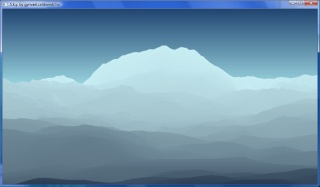static float fg_end   = { 200.0f, 237.0f, 243.0f };  static float fg_diff  = { (fg_end - fg_start) / (float)(HMAP_DEPTH),                        (fg_end - fg_start) / (float)(HMAP_DEPTH),                        (fg_end - fg_start) / (float)(HMAP_DEPTH) };  [...]  // Get the height  float max_y = hmap[idx];  z = idx / HMAP_WIDTH;  // Calc color  Vector3D color = Vector3D(    (fg_start + fg_diff * (float)z) / 255.0,    (fg_start + fg_diff * (float)z) / 255.0,    (fg_start + fg_diff * (float)z) / 255.0    );  Vector3D vp_color = color;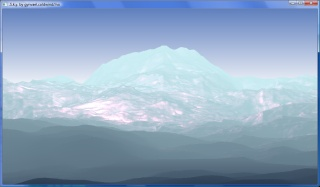// Sun light  // Setup vectors  Vector3D vp = Vector3D( (float)x, (float)max_y, (float)z );  Vector3D L = sun_pos - vp;  L.Norm();  Vector3D N = NormalMap[idx];  float per = 0;  per = ((float)(z/* - 2*HMAP_WIDTH/4*/) / (float)HMAP_DEPTH);  per *= per;  per *= per;  per *= per;  per = 1.0f - per;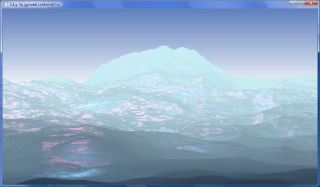// Diffusive  float dot;  dot = L.Dot(NormalMap[idx]);  if (dot < 0.0)  {    float diff = -dot * 0.1f * per; // Play with this to get nice effects    color += (sun_color * vp_color) * diff;  }  // Diffusive 2  if (dot < -0.2)  {    float diff = dot*dot*dot*dot*dot*dot * 0.5f * per; // Play with this to get nice effects    color += sun_color * diff;  }  // Specular  Vector3D R = L -  N * L.Dot(N) * 2.0l;  Vector3D Cs = Vector3D(0, max_y, max_y);  Cs.Norm();  dot = Cs.Dot(R);  if (dot < 0.0)  {    dot = pow(dot, 40.0);    float spec = dot * (0.2f * per); // Play with this to get nice effects    color += sun_color2 * spec;  }  // Check  if(color.arr > 1.0f) color.arr = 1.0f;  if(color.arr > 1.0f) color.arr = 1.0f;  if(color.arr > 1.0f) color.arr = 1.0f;  // Write pixel color  // Uncomment other shaders to see the normals or the dot product  // Shader - full  img[x + y * WIDTH].b = (unsigned char)(color.arr * 255.0f);  img[x + y * WIDTH].g = (unsigned char)(color.arr * 255.0f);  img[x + y * WIDTH].r = (unsigned char)(color.arr * 255.0f);`

Changing the color of the sky and terrain can lead to some more or less interesting results: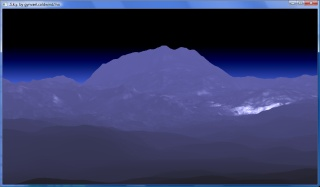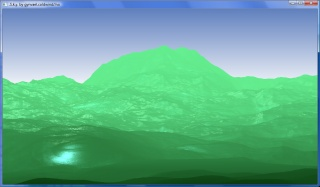The images can be GIMPed too ;D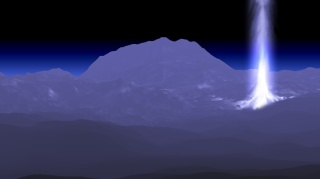And that is all for today. As an "epilogue" - some links to code and movies:

Sky.zip (6mb) - source using SDL (SDL required, 1.2.12), Linux/Windows/MacOSX, bundled with heightmap
Sky_SDL.zip (6mb) - source using SDL (SDL required, 1.2.12) + Windows executable, bundled with heightmap
Sky_Gdi.zip (10kb) - source using WinAPI (GDI), Windows only, no heightmap
sky.avi (1mb) - a video

How to compile:
` Windows Vista SP1 (g++ Sky.cpp Vector3D.cpp -lSDL) MacOSX 10.5.5     (g++ `sdl-config --cflags` Sky.cpp Vector3D.cpp `sdl-config --libs`) Ubuntu 8.10       (g++ `sdl-config --cflags` Sky.cpp Vector3D.cpp `sdl-config --libs`)`

2009-01-24 06:25:49 = Jarek
{
Under Mac and SDL installed using Fink you need to use a little trick in order to compile:

JH-Mac:Sky jarek\$ g++ `sdl-config --cflags` Sky.cpp Vector3D.cpp `sdl-config --libs`
Sky.cpp:47:21: error: SDL/SDL.h: No such file or directory
Sky.cpp:524: error: expected initializer before 'RenderWorker'
Sky.cpp:551: error: 'SDL_Surface' was not declared in this scope
Sky.cpp:551: error: 'Screen' was not declared in this scope
Sky.cpp:552: error: expected ',' or ';' before '{' token
Sky.cpp: In function 'int main(int, char**)':
Sky.cpp:662: error: 'SDL_INIT_VIDEO' was not declared in this scope
Sky.cpp:662: error: 'SDL_INIT_NOPARACHUTE' was not declared in this scope
Sky.cpp:662: error: 'SDL_Init' was not declared in this scope
Sky.cpp:665: error: 'SDL_Surface' was not declared in this scope
Sky.cpp:665: error: 'Screen' was not declared in this scope
Sky.cpp:666: error: 'SDL_HWSURFACE' was not declared in this scope
Sky.cpp:666: error: 'SDL_SetVideoMode' was not declared in this scope
Sky.cpp:673: error: 'SDL_WM_SetCaption' was not declared in this scope
Sky.cpp:679: error: 'SDLCALL' was not declared in this scope
Sky.cpp:679: error: expected primary-expression before 'int'
Sky.cpp:679: error: expected `)' before 'int'
Sky.cpp:685: error: 'SDL_Event' was not declared in this scope
Sky.cpp:685: error: expected `;' before 'Ev'
Sky.cpp:688: error: 'Ev' was not declared in this scope
Sky.cpp:689: error: 'SDL_PollEvent' was not declared in this scope
Sky.cpp:694: error: 'SDL_KEYDOWN' was not declared in this scope
Sky.cpp:695: error: 'SDLK_ESCAPE' was not declared in this scope
Sky.cpp:700: error: 'SDL_MOUSEMOTION' was not declared in this scope
Sky.cpp:706: error: 'SDL_QUIT' was not declared in this scope
Sky.cpp:713: error: 'SDL_Delay' was not declared in this scope

JH-Mac:Sky jarek\$ sdl-config --cflags